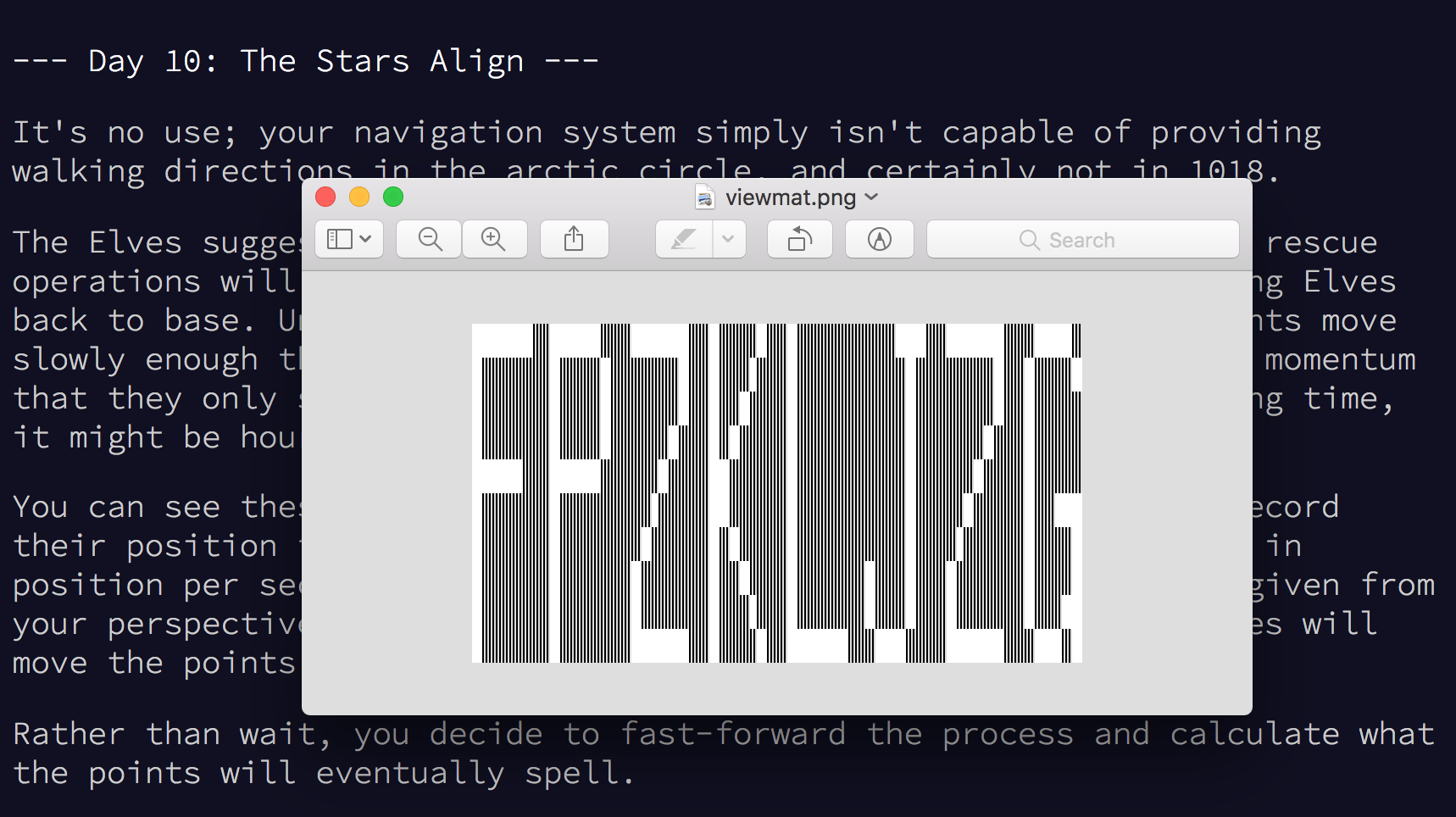The day ten Advent of Code challenge gives us a list of points and velocity pairs. Each point is updated by its corresponding velocity every second. At some point in time, these points will converge and spell out a message. Our task is to find that message using the J programming language!

As usual, we’ll start by loading our input and massaging it into a form we can work with:

``````    replacements =. 'position=<';'';',';'';'> velocity=<';' ';'>';'';'-';'_'
input =. ". > cutopen replacements rplc~ (1!:1) < path
``````

I’m using a trick I learned from `zxw` on Twitter to easily replace and reformat the data before passing it into the “numbers” verb (`".`).

Next let’s write a `tick` verb that updates each point with its corresponding velocity. We’ll also keep track of the maximum spread between Y coordinate values and return that as the second value in a boxed tuple along with our next set of points and velocities:

``````    tick =. 3 : 0
input =. 0 {:: y
prev =. 1 {:: y
next =. +/"1 |:"2 (2 2 \$ ])"1 input
max =. >./ 1 {"1 next
min =. <./ 1 {"1 next
diff =. | max - min
if. diff < prev do.
(next (0 1})"1 input);diff
else.
y
end.
)
``````

Notice that if applying the next `tick` results in a lower spread, we return the new values. Otherwise, we return the old values. This means we can “converge” (`^:_`) on a result for this verb. The result we converge on will be the set of points with the lowest spread in the vertical dimension.

It turns out that this is our answer!

We can use J’s `viewmat` library to quickly visualize our answer (after some more rotating and massaging):

``````    load 'viewmat'

to_mat =. 3 : 0
min_x =. <./ 0 {"1 y
min_y =. <./ 1 {"1 y
max_x =. >./ 0 {"1 y
max_y =. >./ 1 {"1 y
coords =. 0 1 {"1 y
coords =. (min_x,min_y) -~"1 coords
mat =. ((1 + | max_y - min_y),(1 + | max_x - min_x)) \$ 0
)

viewmat to_mat 0 {:: tick^:_ input;_
``````The stars align.

## Part Two

Part two turned out to be a simple modification of our part one solution. The challenge asked us to return how many ticks we went through before we found our message.

All we need to do to figure this out is to add a third element to the tuple we pass in and out of `tick` that holds an incrementing count:

``````    tick =. 3 : 0
input =. 0 {:: y
prev =. 1 {:: y
count =. 2 {:: y
next =. +/"1 |:"2 (2 2 \$ ])"1 input
max =. >./ 1 {"1 next
min =. <./ 1 {"1 next
diff =. | max - min
if. diff < prev do.
(next (0 1})"1 input);diff;(count + 1)
else.
y
end.
)

2 {:: tick^:_ input;_;0
``````

The answer to our challenge is the value of this final count.